# 早晨之星K线形态测试和代码版本汇总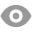952 |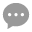11 |Denny | 2020-4-7 23:17:00 | 显示全部楼层 |阅读模式
 1) 早晨之星的特征形态条件1：形态出现在下跌行情中。量化定义：第1根K线收盘价<20日均线形态条件2：第1根K线是下跌棒体量化定义：第1根K线实体>K线整体的0.5倍，并且收盘价<前一天收盘价形态条件3：第2根K线是下跌星线量化定义：第2根K线实体K线整体的0.5倍，并且最高价是整个形态的最高价 2) 测试买卖条件：买入条件：早晨之星形态第3根K线处，买入卖出条件：买入后的第二天收盘前，卖出

 测试一代码： //定义仓位 FUND:=10000000; LOTS:=INTPART(FUND*0.8/(CLOSE+FEE));//计算手数，使每次开仓金额都是800W //定义ATR TR:MAX(MAX((H-L),ABS(REF(C,1)-H)),ABS(REF(C,1)-L));//算出(当日最高-当日最低)、(当日最高-昨日收盘)的绝对值、(昨日收盘-当日最低)的绝对值，取这3个值中的最大值为ATR ATR:MA(TR,26);//求26个周期内的TR的简单移动平均 //定义20日均线 MA20:MA(C,20); //定义K线 KXST:=ABS(O-C);//K线实体长度 YXCD:=H-L;//K线影线长度 BT:=KXST>YXCD*0.5;//棒体实体大于影线的0.5倍 XX:=KXST0.5*ATR AND H=HHV(H,3); //2.定义第二天的星线 ZCZX2:=REF(XX,1) AND REF(L,1)=LLV(L,3) AND REF(H,1)0.5*ATR; //4.定义下跌趋势 ZCZX4:=REF(C,2)

 测试二代码： //一、定义仓位 FUND:=10000000; LOTS:=INTPART(FUND*0.8/(CLOSE+FEE));//计算手数，使每次开仓金额都是800W //定义ATR TR:MAX(MAX((H-L),ABS(REF(C,1)-H)),ABS(REF(C,1)-L));//算出(当日最高-当日最低)、(当日最高-昨日收盘)的绝对值、(昨日收盘-当日最低)的绝对值，取这3个值中的最大值为ATR ATR:MA(TR,26);//求26个周期内的TR的简单移动平均 //定义60日均线 MA60:MA(C,60); //定义K线 KXST:=ABS(O-C);//K线实体长度 YXCD:=H-L;//K线影线长度 BT:=KXST>YXCD*0.5;//棒体实体大于影线的0.5倍 XX:=KXST0.5*ATR AND H=HHV(H,3); //2.定义第二天的星线 ZCZX2:=REF(XX,1) AND REF(L,1)=LLV(L,3) AND REF(H,1)0.5*ATR; //4.定义下跌趋势 ZCZX4:=REF(C,2)

 测试三代码： //定义仓位 FUND:=10000000; LOTS:=INTPART(FUND*0.8/(CLOSE+FEE));//计算手数，使每次开仓金额都是800W //定义ATR TR:MAX(MAX((H-L),ABS(REF(C,1)-H)),ABS(REF(C,1)-L));//算出(当日最高-当日最低)、(当日最高-昨日收盘)的绝对值、(昨日收盘-当日最低)的绝对值，取这3个值中的最大值为ATR ATR:MA(TR,26);//求26个周期内的TR的简单移动平均 //定义均线 MA20:MA(C,20); //定义K线 KXST:=ABS(O-C);//K线实体长度 YXCD:=H-L;//K线影线长度 BT:=KXST>YXCD*0.5;//棒体实体大于影线的0.5倍 XX:=KXST0.5*ATR AND H=HHV(H,3); //2.定义第二天的星线 ZCZX2:=REF(XX,1) AND REF(L,1)=LLV(L,3) AND REF(H,1)0.5*ATR; //4.定义下跌趋势 ZCZX4:=REF(C,2)

 测试四代码： //定义仓位 FUND:=10000000; LOTS:=INTPART(FUND*0.8/(CLOSE+FEE));//计算手数，使每次开仓金额都是800W //定义ATR TR:MAX(MAX((H-L),ABS(REF(C,1)-H)),ABS(REF(C,1)-L));//算出(当日最高-当日最低)、(当日最高-昨日收盘)的绝对值、(昨日收盘-当日最低)的绝对值，取这3个值中的最大值为ATR ATR:MA(TR,26);//求26个周期内的TR的简单移动平均 //定义20日均线 MA20:MA(C,20); //定义DMA指标 DDD:MA(CLOSE,10)-MA(CLOSE,50);//短周期收盘价均值与长周期收盘价均值做差 AMA:MA(DDD,10);//10个周期的DDD均值 DDD1:=AMA<0 AND DDD<0; //定义K线 KXST:=ABS(O-C);//K线实体长度 YXCD:=H-L;//K线影线长度 BT:=KXST>YXCD*0.5;//棒体实体大于影线的0.5倍 XX:=KXST0.5*ATR AND H=HHV(H,3); //2.定义第二天的星线 ZCZX2:=REF(XX,1) AND REF(L,1)=LLV(L,3) AND REF(H,1)0.5*ATR; //4.定义下跌趋势 ZCZX4:=REF(C,2)

 测试五代码： //定义仓位 FUND:=10000000; LOTS:=INTPART(FUND*0.8/(CLOSE+FEE));//计算手数，使每次开仓金额都是800W //定义ATR TR:MAX(MAX((H-L),ABS(REF(C,1)-H)),ABS(REF(C,1)-L));//算出(当日最高-当日最低)、(当日最高-昨日收盘)的绝对值、(昨日收盘-当日最低)的绝对值，取这3个值中的最大值为ATR ATR:MA(TR,26);//求N个周期内的TR的简单移动平均 //定义均线 MA20:MA(C,20); //定义K线 KXST:=ABS(O-C);//K线实体长度 YXCD:=H-L;//K线影线长度 BT:=KXST>YXCD*0.5;//棒体实体大于影线的0.5倍 XX:=KXST0.5*ATR AND H=HHV(H,3); //2.定义第二天的星线 ZCZX2:=REF(XX,1) AND REF(L,1)=LLV(L,3) AND REF(H,1)0.5*ATR; //定义早晨之星 ZCZX:=ZCZX1 AND ZCZX2 AND ZCZX3; ZCZX,BK(LOTS);//出现早晨之星买入 BARSBK=1,SP(BKVOL);//1日后卖出

 测试六代码： //定义仓位 FUND:=10000000; LOTS:=INTPART(FUND*0.8/(CLOSE+FEE));//计算手数，使每次开仓金额都是800W //定义ATR TR:MAX(MAX((H-L),ABS(REF(C,1)-H)),ABS(REF(C,1)-L));//算出(当日最高-当日最低)、(当日最高-昨日收盘)的绝对值、(昨日收盘-当日最低)的绝对值，取这3个值中的最大值为ATR ATR:MA(TR,26);//求26个周期内的TR的简单移动平均 //定义常规K线 KXST:=ABS(O-C); SYXCD:=H-MAX(O,C);//上影线长度 XYXCD:=MIN(O, C)-L;//下影线长度 YXCD:=H-L;//影线长度 BT:=KXST>YXCD*0.5;//棒体 //定义十字星 SZXST:=KXST0 AND XYXCD>0;//十字星定义 //早晨之星 //第三天的上涨棒体 ZCZX1:=BT AND YXCD>0.5*ATR AND C=HHV(C,3) AND H>=HHV(H,2); //第二天的十字星 ZCZX2:=REF(SZX,1) AND REF(L,1)=LLV(L,3) AND REF(H,1)0.5*ATR; //定义早晨之星 ZCZX:=ZCZX1 AND ZCZX2 AND ZCZX3; ZCZX,BK(LOTS);//出现早晨之星买入 BARSBK=1,SP(BKVOL);//1日后卖出

 测试代码： //定义仓位 FUND:=10000000; LOTS:=INTPART(FUND*0.04/(CLOSE+FEE));//计算手数，使每次开仓金额都是800W //定义ATR TR:MAX(MAX((H-L),ABS(REF(C,1)-H)),ABS(REF(C,1)-L));//算出(当日最高-当日最低)、(当日最高-昨日收盘)的绝对值、(昨日收盘-当日最低)的绝对值，取这3个值中的最大值为ATR ATR:MA(TR,26);//求26个周期内的TR的简单移动平均 //定义常规K线 KXST:=ABS(O-C); SYXCD:=H-MAX(O,C);//上影线长度 XYXCD:=MIN(O, C)-L;//下影线长度 YXCD:=H-L;//影线长度 BT:=KXST>YXCD*0.5;//棒体 //定义十字星 SZXST:=KXST0 AND XYXCD>0;//十字星定义 //早晨之星 //第三天的上涨棒体 ZCZX1:=BT AND YXCD>0.5*ATR AND C=HHV(C,3) AND H>=HHV(H,2); //第二天的十字星 ZCZX2:=REF(SZX,1) AND REF(L,1)=LLV(L,3) AND REF(H,1)0.5*ATR; //定义早晨之星 ZCZX:=ZCZX1 AND ZCZX2 AND ZCZX3; ZCZX,BK(LOTS);//出现早晨之星买入 BARSBK=1,SP(BKVOL);//1日后卖出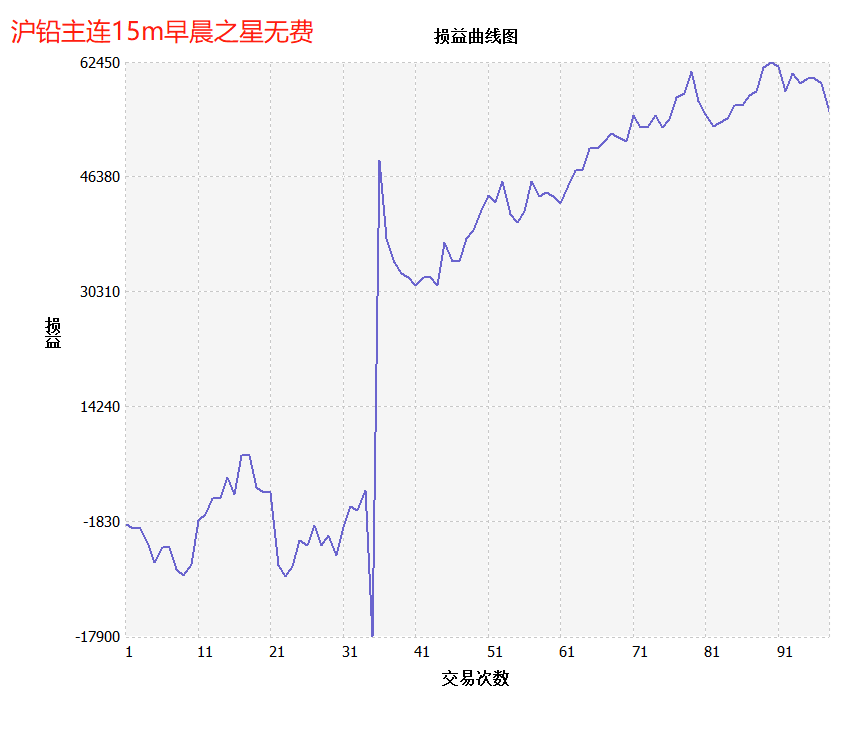测试品种：沪铅主连；测试周期：15分钟K线 胜率58.16%，盈亏比：1.36；胜率盈亏：0.3726；交易次数：98次；最大回撤比：0.25%； 测试周期数：58092；空仓周期数：57994；持仓天数：98；单位效率：0.00003%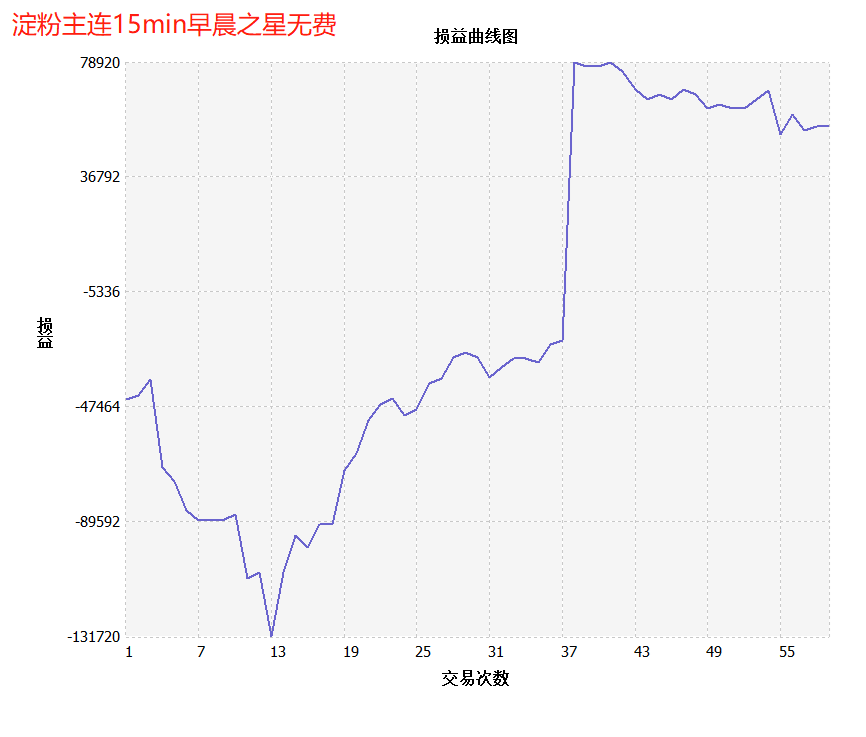测试品种：淀粉主连；测试周期：15分钟K线 胜率62.71%，盈亏比：0.92；胜率盈亏：0.2040；交易次数：59次；最大回撤比：1.32%； 测试周期数：24754；空仓周期数：24695；持仓天数：59；单位效率：0.00005%

 您需要登录后才可以回帖 登录 | 立即注册 本版积分规则 回帖后跳转到最后一页87主题 1226积分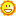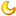1226